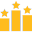### Keresés

Keresendő kifejezés:

### Toplista• betöltés...

Segítség!

Ahhoz, hogy mások kérdéseit és válaszait megtekinthesd, nem kell beregisztrálnod, azonban saját kérdés kiírásához ez szükséges!

### Maths in english

96
Jelenleg 1 felhasználó nézi ezt a kérdést.
0
Középiskola / Matematika

### Válaszok

1
First let's see a kind of definition: (if you find it trivial it is not required...)
The distance between a point (a single point) and a circle is the shortest possible line segment that connects the point and the circle. It can be constructed this way:
- draw a line between the point P and the centre of the circle
- if the point is inside the circle, extend the line to reach the circle
- the line intersects the circle in points Q and R
- the line segment to be contructed is the shortest of QP or RP

This distance can be calculated like this: (this is also not required for this exercise, but may shed some light...)
- calculate the distance between the point and the centre of the cirlce
bb"And now the real solution:"
- Select any 3 points from the 4 ones and construct a circle through them. The centre of this circle is O, the radius is r_1.
- Then construct a circle through the fourth point from the same centre O, the radius is r_2
- The solution will be a third circle centered at O with radius (r_1+r_2)/2
Of course it only works if the fourth point is not lying on the first circle. In that case (so when the 4 points lie on a scircle) there are infinte number of solutions that are all centered at O.
Normally the four points are not on a single circle; then there are ((4),(3))=4 different ways of choosing 3 points from the 4 ones, so you get 4 solutions.